Mathematics Introduction of Integrals and Geometrical interpretation
Click for Only Video

### Topic covered

color {red} star Introduction
color {red} star Integration as an Inverse Process of Differentiation
color {red} star Formulae Of integration
color {red} starGeometrical interpretation of indefinite integral
color {red} star Properties of indefinite integral

### Introduction

\color{green} ✍️ Process of finding anti derivatives is called integration .
\color{green} ✍️ There are two forms of the integrals, e.g., indefinite and definite integrals, which together constitute the Integral Calculus.

### Integration as an Inverse Process of Differentiation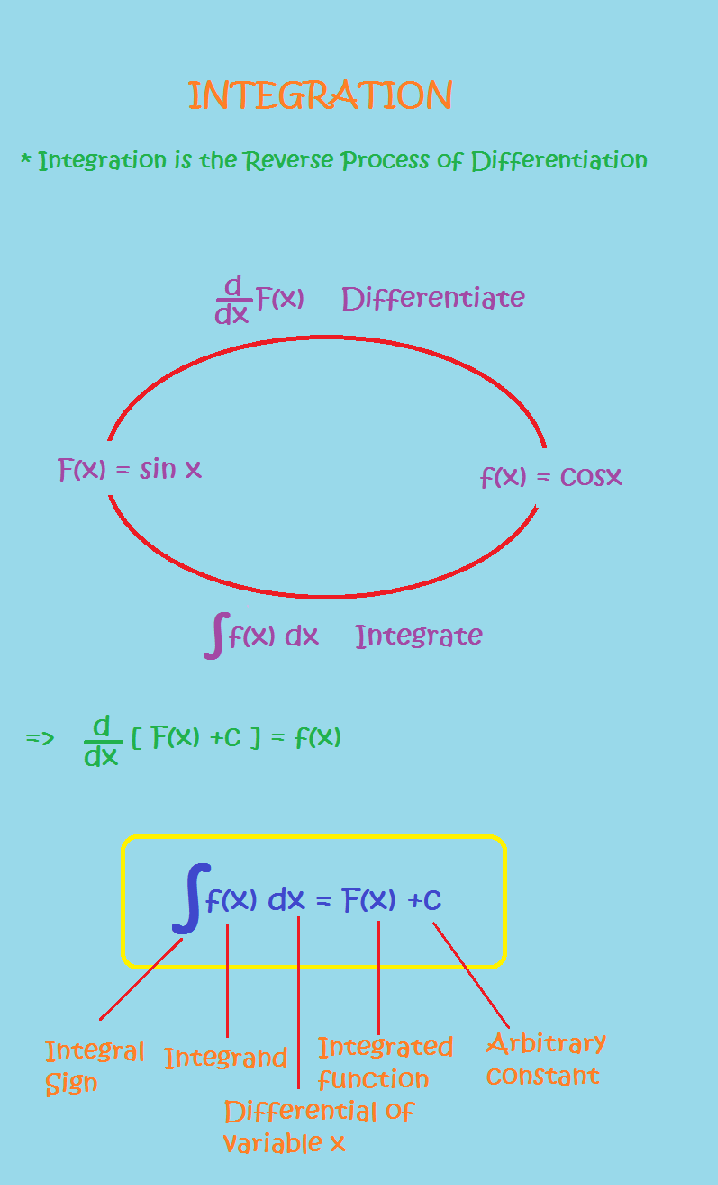\color{green} ✍️ Integration is the inverse process of differentiation.

\color{green} ✍️ Instead of differentiating a function, we are given the derivative of a function and asked to find its primitive, i.e., the original function. Such a process is called integration or anti differentiation.

\color{green} ✍️ If there is a function F such that d/(dx) F(x) = f(x) , AA x ∈ I (interval), then for any arbitrary real number C, (also called constant of integration)

color{orange}{d/(dx) [F(x) +C ] = f(x) , x ∈ I"

\color{green} ✍️ A new symbol, namely, ∫ f (x) dx which will represent the entire class of"
\color{green}" anti derivatives read as the indefinite integral of f with respect to x

Symbolically, we write color{orange} (∫ f (x) dx = F (x) + C.)

Notation Given that  (dy)/(dx)= f(x) , we write y = ∫ f (x) dx .

- we mention below the following symbols/terms/phrases with their meanings as given in the Table.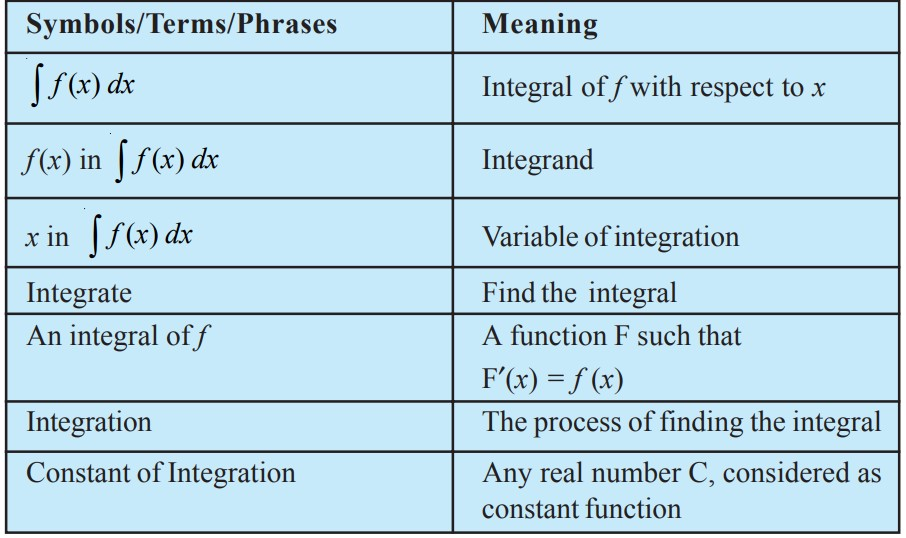### Formulae of Integration(Must remember)

Derivatives Integrals (Anti Derivative )
(i) d/(dx) ( (x^(n+1))/(n+1) ) = x^n Particularly d/(dx)x = 1 int x^n dx = x^(n +1) /(n+1) + C , n != -1  intdx = x +C
(ii) color{blue} {d/(dx) (sinx) = cos x} ; color{green} { int cos x dx = sinx + C
(iii) color{blue} {d/(dx) ( - cos x ) = sinx} ; color{green} {int sinx dx = - cos x + C}
(iv) color{blue} {d/(dx) (tan x) = sec^2x} ; color{green} { int sec^2 x dx = tan x +C}
(v) color{blue} {d/(dx) (-cot x ) = cosec^2x} ;  color{green} { int cosec^x dx = - cot x +C}
(vi) color{blue} { d/(dx) (sec x ) = sec x tan x } ;  color{green} {int sec tan x dx = sec x + C}
(vii) color{blue} {d/(dx) (- cosec x ) = cosec x cot x }; color{green} {int cosec x cot x dx = - cosec x + C}
(viii) color{blue} {d/(dx) (sin^-1 x ) = 1/ sqrt (1 -x^2)} color{green} {int (dx)/sqrt(1 -x^2) = sin^(-1) x + C }
(ix) color{blue} {d/(dx) ( - cos^(-1) x ) = 1/ sqrt (1 -x^2)}  color{green} {int (dx)/sqrt(1 -x^2) = - cos^(-1) x + C}
(x) color{blue} {d/(dx) ( tan^(-1) x ) = 1/ (1 +x^2)} color{green} { int (dx)/sqrt(1 +x^2) = tan^(-1) x + C}
(xi) color{blue} { d/(dx) ( - cot ^(-1) x ) = 1/ (1 +x^2)} color{green} {int (dx)/sqrt(1 +x^2) = -cot^(-1) x + C}
(xiii) color{blue} {d/(dx) (sec^-1 x) = 1/(x sqrt(x^2 -1))} ; color{green} { int (dx)/(xsqrt(x^2 -1) ) = sec^-1 x +C}
(xiii) color{blue} {d/(dx) ( - cosec^-1 x) = 1/(x sqrt(x^2 -1))} ; color{green} { int (dx)/(xsqrt(x^2 -1) ) = -cosec^-1 x +C}
(xiv) color{blue} {g/(dx) (e^x) = e^x} ; color{green} {int e^x dx = e^x +C}
(xv) color{blue} {d/(dx) ( log | x | ) = 1/x} ;  color{green} {int 1/x dx = log |x| +C}
(xvi) color{blue} {d/(dx) ( a^x/ (log a) ) = a^x} ; color{green} {int a^x dx = a^x/(log a ) +C}

### Geometrical interpretation of indefinite integral● Let f (x) = 2x, Then color{orange}(∫ f (x) dx = x^2 + C) . For different values of C, we get different integrals. But these integrals are very similar geometrically.

● y = x^2 + C, where C is arbitrary constant, represents a family of integrals. By assigning different values to C, we get different members of the family.

● Clearly, for C = 0, we obtain y = x^2 , a parabola with its vertex on the origin. The curve y = x^2 + 1 for C = 1 is obtained by shifting the parabola y = x^2 one unit along y-axis in positive direction. For C = – 1, y = x^2 – 1 is obtained by shifting the parabola y = x^2
one unit along y-axis in the negative direction and so on.

● So color{orange}{∫ f (x) dx = x^2 + C}" represents a family of curves." The different values of C will correspond to different members of this family and these members can be obtained by shifting any one of the curves parallel to itself.

● "This is the geometrical interpretation of indefinite integral."

### Properties of indefinite integral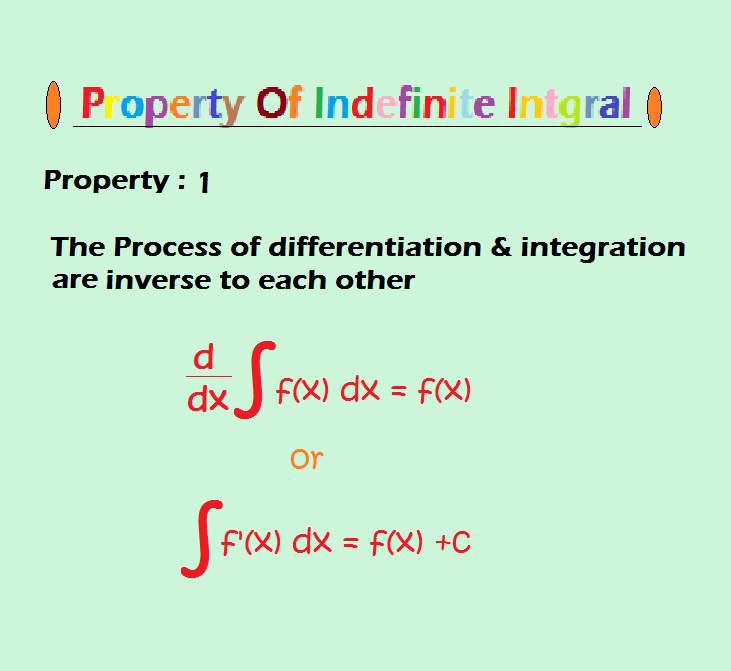"Property 1 :"

color{blue}"The process of differentiation and integration are inverses of each other"

color{orange} {=> d/(dx) ∫ f(x) dx = f(x)} and  color{orange} {∫ f' (x) dx = f(x) +C} ,

where C  is any arbitrary constant.

color{red}{ul{" Proof :" Let F be any anti derivative of f, i.e.,

color{orange} {d/(dx) F(x) = f(x)}

Then  color{orange} {∫ f (x) dx = F(x) +C}

therefore color{orange} {d/(dx) ∫ f(x) dx = d/(dx) (F(x) +C )}

 color{orange} { = d/(dx) F(x) = f(x)}

Similarly, we note that

color{orange} {f'(x) = d/(dx) f(x)} and hence color{orange} {∫ f ′(x) dx = f (x) + C}

where C is arbitrary constant called constant of integration.

### Property 2 :

color{blue}"Two indefinite integrals with the same derivative lead to the same family of curves and so they are equivalent. "

color{red}{ul{" Proof :" Let f and g be two functions such that

color{orange} {d/(dx) ∫ f(x) dx = d/(dx) ∫ g (x) dx}

or color{orange} {d/(dx) [ ∫ f(x) dx- ∫ g(x) dx ] = 0}

Hence  color{orange} {∫ f(x) dx - ∫ g (x) dx = C} , where C is any real number

or  color{orange} {∫ f (x) dx = ∫ g (x) dx + C}

So the families of curves  color{green} { ∫ f(x) dx +C_1 ,C_1 ∈ R }

and  color{green} { ∫ g(x) dx +C_2, C_2 ∈ R }are identical

Hence, in this sense , color{orange} {∫ f (x) dx} and  color{orange} {∫ g (x) dx}  are equivalent.

### Property 3 :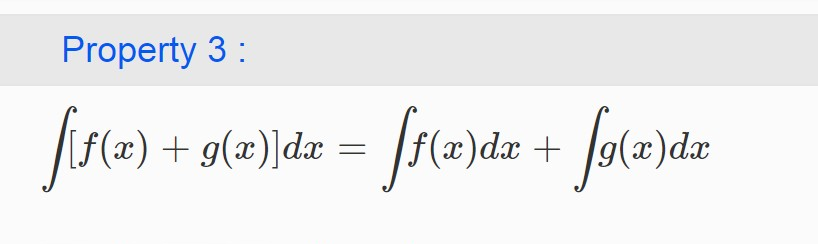color{blue}( ∫ [ f(x) +g(x) ] dx = ∫ f(x) dx + ∫ g(x) dx)

color{red}{ul{" Proof :" By Property (I), we have

color{orange} {d/(dx) [ ∫ [ f(x) + g(x)] dx ] = f(x) + g(x)} ........(1)

On the otherhand, we find that

color{orange} {d/(dx) [ ∫ f(x) dx + ∫ g(x) dx ] = d/(dx) ∫ f(x) dx + d/(dx) ∫ g(x) dx}

color{orange} {= f(x) + g(x)} ........(2)

Thus, in view of Property (II), it follows by (1) and (2) that

color{orange} { ∫ ( f(x) +g(x) ) dx = ∫ f(x) dx + ∫ g(x) dx}

### Property 4 :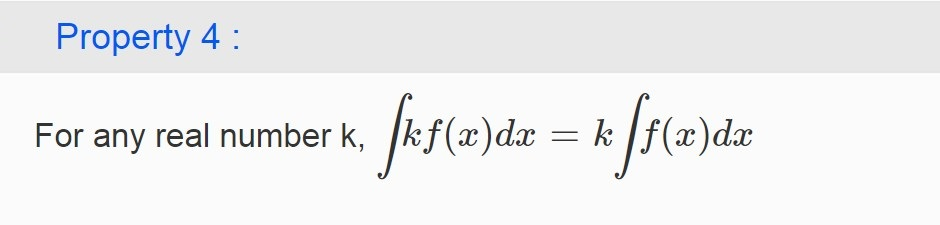For any real number k, color{blue}( ∫ k f(x) dx= k ∫ f(x) dx)

Proof By the Property (I), color{orange} {d/(dx) ∫ k f(x) dx = k f(x)}

Also color{orange} {d/(dx) [ k ∫ f(x) dx ] = k d/(dx) ∫ f(x) dx = k f(x)}

Therefore, using the Property (II), we have  color{orange} {∫ f(x) dx = k ∫ f(x) dx} .

### Property 5 :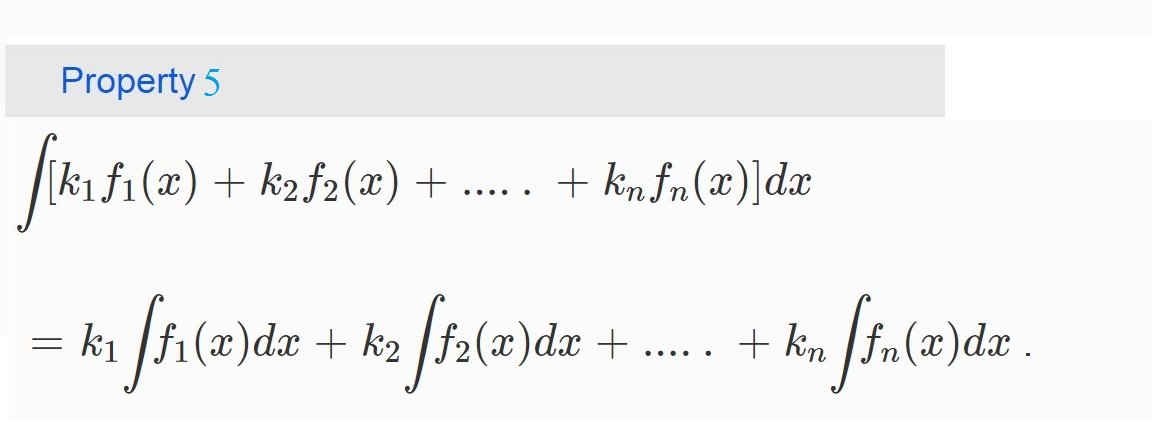- By Properties (III) and (IV) can be generalised to a finite number of functions color{green} {f_1, f_2, ..., f_n} and the real numbers, color{green} {k_1, k_2, ..., k_n} giving

color{orange} { ∫ [k_1 f_1 (x) + k_2 f_2 (x) + .....+ k_n f_n (x) ] dx}

color{orange} { = k_1 ∫ f_1 (x) dx + k_2 ∫ f_2(x) dx + .....+ k_n ∫ f_n (x) dx} .

### Examples

Q 3175278166Write an anti derivative for each of the following functions using the
method of inspection:

(i) cos 2x

(ii) 3x^2 + 4x^3

(iii) 1/x , x ≠ 0
Class 12 Chapter 7 Example 1Solution:

(i) We look for a function whose derivative is cos 2x. Recall that

d/(dx) sin 2x = 2 cos 2x

or cos 2x = 1/2 d/(dx) (sin 2x ) = d/(dx) (1/2 sin 2x )

Therefore, an anti derivative of cos 2x is 1/2 sin 2x .

(ii) We look for a function whose derivative is 3x^2 + 4x^3. Note that

d/(dx) (x^3 + x^4 ) = 3x^2 + 4x^3

Therefore, an anti derivative of 3x^2 + 4x^3 is x^3 + x^4.

(iii) We know that

d/(dx) ( log x ) =1/x , x > 0 and d/(dx) [ log (-x) ] = 1/(-x) (-1) = 1/x , x < 0

Combining above, we get d/(dx) ( log |x| ) = 1/x , x ≠ 0

Therefore,  int 1/x dx = log |x| is one of the anti derivatives of 1/x .
Q 3115278169Find the following integrals:

(i) int (x^3-1)/(x^2) dx

(ii) int (x^2/3 +1) dx

(iii) int ( x^3/2 + 2 e^x -1/x ) dx
Class 12 Chapter 7 Example 2Solution:

(i) We have

 int (x^3-1)/( x^2) dx = int x dx - int x^(-2) dx (by Property V)

= ( ( x^(1+1) )/(1+1) + C_1 ) - ( ( x^(-2+1) )/(-2+1) + C_2 ) ; C_1 , C_2 are constants of integration

= x^2/2 + C_1 - ( x^(-1))/( -1) -C_2 = x^2/2 +1/x +C_1 -C_2

= (x^2)/2 +1/x +C , where C = C_1 – C_2 is another constant of integration

(ii) We have

int (x^2/3 +1) dx = int x^2/3 dx+ int dx

 = (x^(2/3 +1) )/(2/3 +1) + x + C= 3/5 x^5/3 + x+ C

(iii) We have

int (x^3/2 + 2 e^x - 1/x) dx = int x^3/2 dx + int 2 e^x dx - int 1/x dx

= (x^(3/2+1) )/( 3/2+1) + 2 e^x - log | x| + C

= 2/5 x^5/2 + 2 e^x -log |x| + C
Q 3115378260Find the following integrals:

(i) int (sin x + cos x) dx

(ii) int cosec x (cosec x + cot x) dx

(iii) int (1- sin x )/( cos^2 x) dx
Class 12 Chapter 7 Example 3Solution:

(i) We have

∫(sin x + cos x) dx = ∫sin x dx + ∫cos x dx

= – cos x + sin x + C

(ii) We have

∫(cosec x (cosec x + cot x) dx = ∫cosec2 x dx + ∫cosec x cot x dx

= – cot x – cosec x + C

(iii) We have

 int (1- sin x )/(cos^2 x) = int 2/(cos^2 x) dx - int (sin x)/(cos^2 x) dx

 = int sec^2 x dx - int tan x sec x dx

= tan x – sec x + C
Q 3125378261Find the anti derivative F of f defined by f (x) = 4x^3 – 6 , where F (0) = 3
Class 12 Chapter 7 Example 4Solution:

One anti derivative of f (x) is x^4 – 6x since

d/(dx) ( x^4 -6x ) = 4x^3 - 6

Therefore, the anti derivative F is given by

F(x) = x^4 – 6x + C, where C is constant.

Given that F(0) = 3, which gives,

3 = 0 – 6 × 0 + C or C = 3

Hence, the required anti derivative is the unique function F defined by

F(x) = x^4 – 6x + 3.

### Remarks

(i) We see that if F is an anti derivative of f, then so is F + C, where C is an constant. Thus, if we know one anti derivative F of a function f, we can write down an infinite number of anti derivatives of f by adding any constant to F expressed by F(x) + C, C ∈ R. In applications, it is often necessary to satisfy an additional condition which then determines a specific value of C giving unique anti derivative of the given function.

(ii) Sometimes, F is not expressible in terms of elementary functions viz., polynomial, logarithmic, exponential, trigonometric functions and their inverses etc. We are therefore blocked for finding ∫f (x) dx. For example, it is not possible to find ∫e^((-x)^2) dx by inspection since we can not find a function whose derivative is e^((-x)^2).

(iii) When the variable of integration is denoted by a variable other than x, the integral formulae are modified accordingly. For instance

int y^4 dy = y^5/5 + c